# 68 Results

View
Selected filters:
• U.C. BerkeleyOnly Sharing Permitted
CC BY-NC-ND
Rating

يهدف هذا الدرس إلى مساعدة المعلمين على تقييم مدى نجاح الطلاب في تصوِّر المقاطع العرضية ثنائية الأبعاد الممثلة في الكائنات ثلاثية الأبعاد. يساعدك الدرس خصوصًا على تحديد الطلاب الذين يعانون من صعوبات في ادراك المقاطع العرضية ثنائية الأبعاد على طول النقاط المختلفة على مسطح ممثل في جسم ثلاثي الأبعاد ورسمها ومساعدة أولئك الطلاب.

نوع المادة:
التقييم
Lesson Plan
Provider:
Shell Center for Mathematical Education
U.C. Berkeley
Provider Set:
Mathematics Assessment Project (MAP)
04/26/2013Only Sharing Permitted
CC BY-NC-ND
Rating

يهدف هذا الدرس لمساعدة المعلمين على تقييم مدى نجاح الطلاب في: العمل مع مفاهيم التطابق والتشابه، بما في ذلك تحديد الأضلاع المتناظرة والزوايا المتطابقة في المثلثات وبينها؛ وتحديد أهمية وجود المثال المضاد وفهمها؛ اثبات البراهين في سياق هندسي و تقييمها.

نوع المادة:
التقييم
Lesson Plan
Provider:
Shell Center for Mathematical Education
U.C. Berkeley
Provider Set:
Mathematics Assessment Project (MAP)
04/26/2013Only Sharing Permitted
CC BY-NC-ND
Rating

يهدف هذا الدرس لمساعدتك في تقييم مدى نجاح الطلاب باستخدام الخصائص الهندسية من أجل حل المشاكل. على وجه الخصوص، سوف تدعمكم في تحديد ومساعدة الطلاب الذين لديهم الصعوبات التالية: حل المشاكل المتعلقة باستخدام قياسات الزوايا الداخلية للمضلعات؛ وحل المشاكل المتعلقة باستخدام قياسات الزوايا الخارجية للمضلعات.

نوع المادة:
التقييم
Lesson Plan
Provider:
Shell Center for Mathematical Education
U.C. Berkeley
Provider Set:
Mathematics Assessment Project (MAP)
04/26/2013Only Sharing Permitted
CC BY-NC-ND
Rating

يهدف هذا الدرس لمساعدة المعلمين على تقييم مدى نجاح الطلاب في إنشاء المعادلات الخطية وحلها. على وجه الخصوص، يساعدك الدرس على تحديد الطلاب الذين لديهم الصعوبات الآتية ومساعدتهم: حل عادلات بمتغير واحد وحل المعادلات الخطية بأكثر من طريقة واحدة.

نوع المادة:
التقييم
Lesson Plan
Provider:
Shell Center for Mathematical Education
U.C. Berkeley
Provider Set:
Mathematics Assessment Project (MAP)
04/26/2013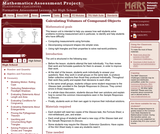Only Sharing Permitted
CC BY-NC-ND
Rating

This lesson unit is intended to help teahcers assess how well students solve problems involving measurement, and in particular, to identify and help students who have the following difficulties; computing measurements using formulas; decomposing compound shapes into simpler ones; using right triangles and their properties to solve real-world problems.

Material Type:
Assessment
Lesson Plan
Provider:
Shell Center for Mathematical Education
U.C. Berkeley
Provider Set:
Mathematics Assessment Project (MAP)
04/26/2013Only Sharing Permitted
CC BY-NC-ND
Rating

This lesson unit is intended to help teachers assess how well students are able to classify solutions to a pair of linear equations by considering their graphical representations. In particular, this unit aims to help teachers identify and assist students who have difficulties in: using substitution to complete a table of values for a linear equation; identifying a linear equation from a given table of values; and graphing and solving linear equations.

نوع المادة:
التقييم
Lesson Plan
Provider:
Shell Center for Mathematical Education
U.C. Berkeley
Provider Set:
Mathematics Assessment Project (MAP)
04/26/2013Only Sharing Permitted
CC BY-NC-ND
Rating

This lesson unit is intended to help teachers assess how well students are able to interpret exponential and linear functions and in particular to identify and help students who have the following difficulties: translating between descriptive, algebraic and tabular data, and graphical representation of the functions; recognizing how, and why, a quantity changes per unit intervale; and to achieve these goals students work on simple and compound interest problems.

نوع المادة:
التقييم
Lesson Plan
Provider:
Shell Center for Mathematical Education
U.C. Berkeley
Provider Set:
Mathematics Assessment Project (MAP)
04/26/2013Only Sharing Permitted
CC BY-NC-ND
Rating

This lesson unit is intended to help teachers assess how well students are able to use linear inequalities to create a set of solutions. In particular, the lesson will help teachers identify and assist students who have difficulties in: representing a constraint by shading the correct side of the inequality line; and understanding how combining inequalities affects a solution space.

نوع المادة:
التقييم
Lesson Plan
Provider:
Shell Center for Mathematical Education
U.C. Berkeley
Provider Set:
Mathematics Assessment Project (MAP)
04/26/2013Only Sharing Permitted
CC BY-NC-ND
Rating

This lesson unit is intended to help teachers assess how well students are able to: Select appropriate mathematical methods to use for an unstructured problem; interpret a problem situation, identifying constraints and variables, and specify assumptions; work with 2- and 3-dimensional shapes to solve a problem involving capacity and surface area; and communicate their reasoning clearly.

نوع المادة:
التقييم
Lesson Plan
Provider:
Shell Center for Mathematical Education
U.C. Berkeley
Provider Set:
Mathematics Assessment Project (MAP)
04/26/2013Only Sharing Permitted
CC BY-NC-ND
Rating

This lesson unit is intended to help you assess whether students recognize relationships of direct proportion and how well they solve problems that involve proportional reasoning. In particular, it is intended to help you identify those students who: use inappropriate additive strategies in scaling problems, which have a multiplicative structure; rely on piecemeal and inefficient strategies such as doubling, halving, and decomposition, and have not developed a single multiplier strategy for solving proportionality problems; and see multiplication as making numbers bigger, and division as making numbers smaller.

نوع المادة:
التقييم
Lesson Plan
Provider:
Shell Center for Mathematical Education
U.C. Berkeley
Provider Set:
Mathematics Assessment Project (MAP)
04/26/2013Only Sharing Permitted
CC BY-NC-ND
Rating

This lesson unit is intended to help teachers assess how well students understand the notion of correlation. In particular this unit aims to identify and help students who have difficulty in: understanding correlation as the degree of fit between two variables; making a mathematical model of a situation; testing and improving the model; communicating their reasoning clearly; and evaluating alternative models of the situation.

نوع المادة:
التقييم
Lesson Plan
Provider:
Shell Center for Mathematical Education
U.C. Berkeley
Provider Set:
Mathematics Assessment Project (MAP)
04/26/2013Only Sharing Permitted
CC BY-NC-ND
Rating

This lesson unit is intended to help assess how well students are able to interpret and use scale drawings to plan a garden layout. This involves using proportional reasoning and metric units.

نوع المادة:
التقييم
Lesson Plan
Provider:
Shell Center for Mathematical Education
U.C. Berkeley
Provider Set:
Mathematics Assessment Project (MAP)
04/26/2013Only Sharing Permitted
CC BY-NC-ND
Rating

This lesson unit is intended to help teachers assess how well students are able to: use the Pythagorean theorem to derive the equation of a circle; and translate between the geometric features of circles and their equations.

نوع المادة:
التقييم
Lesson Plan
Provider:
Shell Center for Mathematical Education
U.C. Berkeley
Provider Set:
Mathematics Assessment Project (MAP)
04/26/2013Only Sharing Permitted
CC BY-NC-ND
Rating

This lesson unit is intended to help teachers assess how well students are able to: translate between the equations of circles and their geometric features; and sketch a circle from its equation.

نوع المادة:
التقييم
Lesson Plan
Provider:
Shell Center for Mathematical Education
U.C. Berkeley
Provider Set:
Mathematics Assessment Project (MAP)
04/26/2013Only Sharing Permitted
CC BY-NC-ND
Rating

This lesson unit is intended to help you assess how well students are able to: solve simple problems involving ratio and direct proportion; choose an appropriate sampling method; and collect discrete data and record them using a frequency table.

Material Type:
Assessment
Lecture Notes
Lesson Plan
Teaching/Learning Strategy
Provider:
Shell Center for Mathematical Education
U.C. Berkeley
Provider Set:
Mathematics Assessment Project (MAP)
Author:
http://map.mathshell.org/
04/26/2013Only Sharing Permitted
CC BY-NC-ND
Rating

This lesson unit is intended to help teachers assess how well students are able to: estimate lengths of everyday objects; convert between decimal and scientific notation; and make comparisons of the size of numbers expressed in both decimal and scientific notation.

نوع المادة:
التقييم
Lesson Plan
Provider:
Shell Center for Mathematical Education
U.C. Berkeley
Provider Set:
Mathematics Assessment Project (MAP)
04/26/2013Only Sharing Permitted
CC BY-NC-ND
Rating

This lesson unit is intended to help you assess how well students are able to: Model a situation; make sensible, realistic assumptions and estimates; and use assumptions and estimates to create a chain of reasoning, in order to solve a practical problem.

نوع المادة:
التقييم
Lesson Plan
Provider:
Shell Center for Mathematical Education
U.C. Berkeley
Provider Set:
Mathematics Assessment Project (MAP)
04/26/2013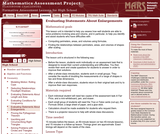Only Sharing Permitted
CC BY-NC-ND
Rating

This lesson unit is intended to help teachers assess how well students are able to solve problems involving area and volume, and in particular, to help you identify and assist students who have difficulties with the following: computing perimeters, areas and volumes using formulas; and finding the relationships between perimeters, areas, and volumes of shapes after scaling.

نوع المادة:
التقييم
Lesson Plan
Provider:
Shell Center for Mathematical Education
U.C. Berkeley
Provider Set:
Mathematics Assessment Project (MAP)
04/26/2013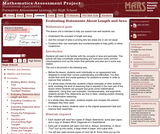Only Sharing Permitted
CC BY-NC-ND
Rating

This lesson unit is intended to help teachers assess how well students can: Understand the concepts of length and area; use the concept of area in proving why two areas are or are not equal; and construct their own examples and counterexamples to help justify or refute conjectures.

نوع المادة:
التقييم
Lesson Plan
Provider:
Shell Center for Mathematical Education
U.C. Berkeley
Provider Set:
Mathematics Assessment Project (MAP)
04/26/2013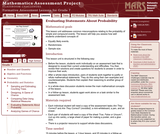Only Sharing Permitted
CC BY-NC-ND
Rating

This lesson unit addresses common misconceptions relating to probability of simple and compound events. The lesson will help you assess how well students understand concepts of: Equally likely events; randomness; and sample sizes.

Material Type:
Assessment
Lesson Plan
Provider:
Shell Center for Mathematical Education
U.C. Berkeley
Provider Set:
Mathematics Assessment Project (MAP)
04/26/2013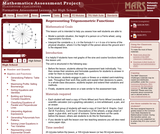Only Sharing Permitted
CC BY-NC-ND
Rating

This lesson unit is intended to help teachers assess how well students are able to: model a periodic situation, the height of a person on a Ferris wheel, using trigonometric functions; and interpret the constants a, b, c in the formula h = a + b cos ct in terms of the physical situation, where h is the height of the person above the ground and t is the elapsed time.

نوع المادة:
التقييم
Lesson Plan
Provider:
Shell Center for Mathematical Education
U.C. Berkeley
Provider Set:
Mathematics Assessment Project (MAP)
04/26/2013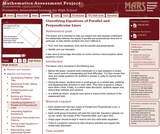Only Sharing Permitted
CC BY-NC-ND
Rating

This lesson unit is intended to help teachers assess how well students are able to understand the relationship between the slopes of parallel and perpendicular lines and, in particular, to help identify students who find it difficult to: find, from their equations, lines that are parallel and perpendicular; and identify and use intercepts. It also aims to encourage discussion on some common misconceptions about equations of lines.

نوع المادة:
التقييم
Lesson Plan
Provider:
Shell Center for Mathematical Education
U.C. Berkeley
Provider Set:
Mathematics Assessment Project (MAP)
04/26/2013Only Sharing Permitted
CC BY-NC-ND
Rating

This lesson unit is intended to help teachers assess how well students are able to understand what the different algebraic forms of a quadratic function reveal about the properties of its graphical representation. In particular, the lesson will help teachers identify and help students who have the following difficulties: understanding how the factored form of the function can identify a graphŐs roots; understanding how the completed square form of the function can identify a graphŐs maximum or minimum point; and understanding how the standard form of the function can identify a graphŐs intercept.

نوع المادة:
التقييم
Lesson Plan
Provider:
Shell Center for Mathematical Education
U.C. Berkeley
Provider Set:
Mathematics Assessment Project (MAP)
04/26/2013Only Sharing Permitted
CC BY-NC-ND
Rating

This lesson unit is intended to help teachers assess how well students are able to: articulate verbally the relationships between variables arising in everyday contexts; translate between everyday situations and sketch graphs of relationships between variables; interpret algebraic functions in terms of the contexts in which they arise; and reflect on the domains of everyday functions and in particular whether they should be discrete or continuous.

نوع المادة:
التقييم
Lesson Plan
Provider:
Shell Center for Mathematical Education
U.C. Berkeley
Provider Set:
Mathematics Assessment Project (MAP)
04/26/2013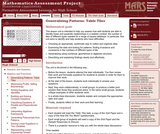Only Sharing Permitted
CC BY-NC-ND
Rating

This lesson unit is intended to help teachers assess how well students are able to identify linear and quadratic relationships in a realistic context: the number of tiles of different types that are needed for a range of square tabletops. In particular, this unit aims to identify and help students who have difficulties with: choosing an appropriate, systematic way to collect and organize data; examining the data and looking for patterns; finding invariance and covariance in the numbers of different types of tile; generalizing using numerical, geometrical or algebraic structure; and describing and explaining findings clearly and effectively.

Material Type:
Assessment
Lesson Plan
Provider:
Shell Center for Mathematical Education
U.C. Berkeley
Provider Set:
Mathematics Assessment Project (MAP)
04/26/2013Only Sharing Permitted
CC BY-NC-ND
Rating

This lesson unit is intended to help you assess how well students working with square numbers are able to: choose an appropriate, systematic way to collect and organize data, examining the data for patterns; describe and explain findings clearly and effectively; generalize using numerical, geometrical, graphical and/or algebraic structure; and explain why certain results are possible/impossible, moving towards a proof.

نوع المادة:
التقييم
Lesson Plan
Provider:
Shell Center for Mathematical Education
U.C. Berkeley
Provider Set:
Mathematics Assessment Project (MAP)
04/26/2013Only Sharing Permitted
CC BY-NC-ND
Rating

This lesson unit is intended to help teachers assess how well students are able to use geometric properties to solve problems. In particular, the lesson will help you identify and help students who have the following difficulties: solving problems by determining the lengths of the sides in right triangles; and finding the measurements of shapes by decomposing complex shapes into simpler ones. The lesson unit will also help students to recognize that there may be different approaches to geometrical problems, and to understand the relative strengths and weaknesses of those approaches.

Material Type:
Assessment
Lesson Plan
Provider:
Shell Center for Mathematical Education
U.C. Berkeley
Provider Set:
Mathematics Assessment Project (MAP)
04/26/2013Only Sharing Permitted
CC BY-NC-ND
Rating

This lesson unit is intended to help you assess how students reason about geometry and, in particular, how well they are able to: use facts about the angle sum and exterior angles of triangles to calculate missing angles; apply angle theorems to parallel lines cut by a transversal; interpret geometrical diagrams using mathematical properties to identify similarity of triangles.

نوع المادة:
التقييم
Lesson Plan
Provider:
Shell Center for Mathematical Education
U.C. Berkeley
Provider Set:
Mathematics Assessment Project (MAP)
04/26/2013Only Sharing Permitted
CC BY-NC-ND
Rating

This lesson unit is intended to help teachers assess how well students are able to interpret percent increase and decrease, and in particular, to identify and help students who have the following difficulties: translating between percents, decimals, and fractions; representing percent increase and decrease as multiplication; and recognizing the relationship between increases and decreases.

نوع المادة:
التقييم
Lesson Plan
Provider:
Shell Center for Mathematical Education
U.C. Berkeley
Provider Set:
Mathematics Assessment Project (MAP)
04/26/2013Only Sharing Permitted
CC BY-NC-ND
Rating

This lesson unit is intended to help teachers assess how well students are able to use geometric properties to solve problems. In particular, it will help you identify and help students who have difficulty: decomposing complex shapes into simpler ones in order to solve a problem; bringing together several geometric concepts to solve a problem; and finding the relationship between radii of inscribed and circumscribed circles of right triangles.

Material Type:
Assessment
Lesson Plan
Provider:
Shell Center for Mathematical Education
U.C. Berkeley
Provider Set:
Mathematics Assessment Project (MAP)
04/26/2013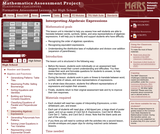Only Sharing Permitted
CC BY-NC-ND
Rating

This lesson unit is intended to help teachers assess how well students are able to translate between words, symbols, tables, and area representations of algebraic expressions. It will help teachers to identify and support students who have difficulty in: recognizing the order of algebraic operations; recognizing equivalent expressions; and understanding the distributive laws of multiplication and division over addition (expansion of parentheses).

نوع المادة:
التقييم
Lesson Plan
Provider:
Shell Center for Mathematical Education
U.C. Berkeley
Provider Set:
Mathematics Assessment Project (MAP)
04/26/2013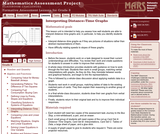Only Sharing Permitted
CC BY-NC-ND
Rating

This lesson unit is intended to help teachers assess how well students are able to interpret distanceĐtime graphs and, in particular, to help you identify students who: interpret distanceĐtime graphs as if they are pictures of situations rather than abstract representations of them; and have difficulty relating speeds to slopes of these graphs.

نوع المادة:
التقييم
Lesson Plan
Provider:
Shell Center for Mathematical Education
U.C. Berkeley
Provider Set:
Mathematics Assessment Project (MAP)
04/26/2013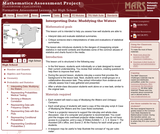Only Sharing Permitted
CC BY-NC-ND
Rating

This lesson unit is intended to help teachers assess how well students are able to: interpret data and evaluate statistical summaries; and critique someone elseŐs interpretations of data and evaluations of statistical summaries. The lesson also introduces students to the dangers of misapplying simple statistics in real-world contexts, and illustrates some of the common abuses of statistics and charts found in the media.

نوع المادة:
التقييم
Lesson Plan
Provider:
Shell Center for Mathematical Education
U.C. Berkeley
Provider Set:
Mathematics Assessment Project (MAP)
04/26/2013Only Sharing Permitted
CC BY-NC-ND
Rating

This lesson unit is intended to help you assess how well students are able to: Perform arithmetic operations, including those involving whole-number exponents, recognizing and applying the conventional order of operations; Write and evaluate numerical expressions from diagrammatic representations and be able to identify equivalent expressions; apply the distributive and commutative properties appropriately; and use the method for finding areas of compound rectangles.

نوع المادة:
التقييم
Lesson Plan
Provider:
Shell Center for Mathematical Education
U.C. Berkeley
Provider Set:
Mathematics Assessment Project (MAP)
04/26/2013Only Sharing Permitted
CC BY-NC-ND
Rating

This lesson unit is intended to help teahcers assess how well students are able to interpret speed as the slope of a linear graph and translate between the equation of a line and its graphical representation.

نوع المادة:
التقييم
Lesson Plan
Provider:
Shell Center for Mathematical Education
U.C. Berkeley
Provider Set:
Mathematics Assessment Project (MAP)
04/26/2013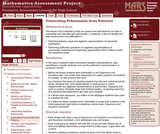Only Sharing Permitted
CC BY-NC-ND
Rating

This lesson unit is intended to help you assess how well students are able to manipulate and calculate with polynomials. In particular, it aims to identify and help students who have difficulties in: switching between visual and algebraic representations of polynomial expressions; and performing arithmetic operations on algebraic representations of polynomials, factorizing and expanding appropriately when it helps to make the operations easier.

نوع المادة:
التقييم
Lesson Plan
Provider:
Shell Center for Mathematical Education
U.C. Berkeley
Provider Set:
Mathematics Assessment Project (MAP)
04/26/2013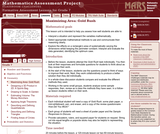Only Sharing Permitted
CC BY-NC-ND
Rating

This lesson unit is intended to help you assess how well students are able to: Interpret a situation and represent the variables mathematically; select appropriate mathematical methods to use; explore the effects on the area of a rectangle of systematically varying the dimensions whilst keeping the perimeter constant; interpret and evaluate the data generated and identify the optimum case; and communicate their reasoning clearly.

نوع المادة:
التقييم
Lesson Plan
Provider:
Shell Center for Mathematical Education
U.C. Berkeley
Provider Set:
Mathematics Assessment Project (MAP)
04/26/2013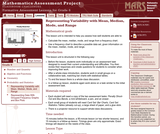Only Sharing Permitted
CC BY-NC-ND
Rating

This lesson unit is intended to help you assess how well students are able to: Calculate the mean, median, mode, and range from a frequency chart; and to use a frequency chart to describe a possible data set, given information on the mean, median, mode, and range.

نوع المادة:
Activity/Lab
التقييم
Lesson Plan
Provider:
Shell Center for Mathematical Education
U.C. Berkeley
Provider Set:
Mathematics Assessment Project (MAP)
المؤلف:
Shell Center Team
01/17/2013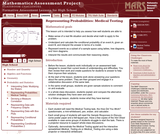Only Sharing Permitted
CC BY-NC-ND
Rating

This lesson unit is intended to help teachers assess how well students are able to: make sense of a real life situation and decide what math to apply to the problem; understand and calculate the conditional probability of an event A, given an event B, and interpret the answer in terms of a model; represent events as a subset of a sample space using tables, tree diagrams, and Venn diagrams; and interpret the results and communicate their reasoning clearly.

نوع المادة:
التقييم
Lesson Plan
Provider:
Shell Center for Mathematical Education
U.C. Berkeley
Provider Set:
Mathematics Assessment Project (MAP)
04/26/2013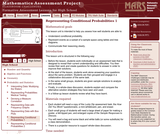Only Sharing Permitted
CC BY-NC-ND
Rating

This lesson unit is intended to help teachers assess how well students are able to: Understand conditional probability; represent events as a subset of a sample space using tables and tree diagrams; and communicate their reasoning clearly.

نوع المادة:
التقييم
Lesson Plan
Provider:
Shell Center for Mathematical Education
U.C. Berkeley
Provider Set:
Mathematics Assessment Project (MAP)
04/26/2013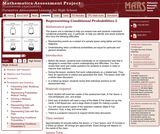Only Sharing Permitted
CC BY-NC-ND
Rating

This lesson unit is intended to help teachers assess how well students understand conditional probability, and, in particular, to help teachers identify and assist students who have the following difficulties: representing events as a subset of a sample space using tables and tree diagrams; and understanding when conditional probabilities are equal for particular and general situations.

نوع المادة:
التقييم
Lesson Plan
Provider:
Shell Center for Mathematical Education
U.C. Berkeley
Provider Set:
Mathematics Assessment Project (MAP)
04/26/2013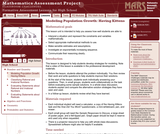Only Sharing Permitted
CC BY-NC-ND
Rating

This lesson unit is intended to help teachers assess how well students are able to: interpret a situation and represent the constraints and variables mathematically; select appropriate mathematical methods to use; make sensible estimates and assumptions; investigate an exponentially increasing sequence; and communicate their reasoning clearly.

نوع المادة:
التقييم
Lesson Plan
Provider:
Shell Center for Mathematical Education
U.C. Berkeley
Provider Set:
Mathematics Assessment Project (MAP)
04/26/2013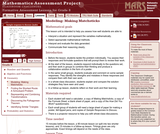Only Sharing Permitted
CC BY-NC-ND
Rating

This lesson unit is intended to help you assess how well students are able to: interpret a situation and represent the variables mathematically; select appropriate mathematical methods; interpret and evaluate the data generated; and communicate their reasoning clearly.

نوع المادة:
التقييم
Lesson Plan
Provider:
Shell Center for Mathematical Education
U.C. Berkeley
Provider Set:
Mathematics Assessment Project (MAP)
04/26/2013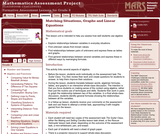Only Sharing Permitted
CC BY-NC-ND
Rating

This lesson unit is intended to help you assess how well students use algebra in context, and in particular, how well students: explore relationships between variables in everyday situations; find unknown values from known values; find relationships between pairs of unknowns, and express these as tables and graphs; and find general relationships between several variables, and express these in different ways by rearranging formulae.

نوع المادة:
التقييم
Lesson Plan
Provider:
Shell Center for Mathematical Education
U.C. Berkeley
Provider Set:
Mathematics Assessment Project (MAP)
04/26/2013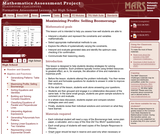Only Sharing Permitted
CC BY-NC-ND
Rating

This lesson unit is intended to help teachers assess how well students are able to: interpret a situation and represent the constraints and variables mathematically; select appropriate mathematical methods to use; explore the effects of systematically varying the constraints; interpret and evaluate the data generated and identify the optimum case, checking it for confirmation; and communicate their reasoning clearly.

نوع المادة:
التقييم
Lesson Plan
Provider:
Shell Center for Mathematical Education
U.C. Berkeley
Provider Set:
Mathematics Assessment Project (MAP)
04/26/2013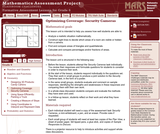Only Sharing Permitted
CC BY-NC-ND
Rating

This lesson unit is intended to help sixth grade teachers assess how well students are able to: Analyze a realistic situation mathematically; construct sight lines to decide which areas of a room are visible or hidden from a camera; find and compare areas of triangles and quadrilaterals; and calculate and compare percentages and/or fractions of areas.

نوع المادة:
التقييم
Lesson Plan
Provider:
Shell Center for Mathematical Education
U.C. Berkeley
Provider Set:
Mathematics Assessment Project (MAP)
04/26/2013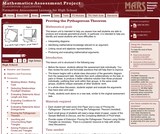Only Sharing Permitted
CC BY-NC-ND
Rating

This lesson unit is intended to help teachers assess how well students are able to produce and evaluate geometrical proofs. In particular, this unit is intended to help you identify and assist students who have difficulties in: interpreting diagrams; identifying mathematical knowledge relevant to an argument; linking visual and algebraic representations; and producing and evaluating mathematical arguments.

نوع المادة:
التقييم
Lesson Plan
Provider:
Shell Center for Mathematical Education
U.C. Berkeley
Provider Set:
Mathematics Assessment Project (MAP)
04/26/2013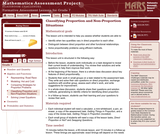Only Sharing Permitted
CC BY-NC-ND
Rating

This lesson unit is intended to help teachers assess whether students are able to: identify when two quantities vary in direct proportion to each other; distinguish between direct proportion and other functional relationships; and solve proportionality problems using efficient methods.

نوع المادة:
التقييم
Lesson Plan
Provider:
Shell Center for Mathematical Education
U.C. Berkeley
Provider Set:
Mathematics Assessment Project (MAP)
04/26/2013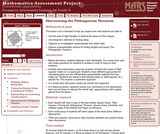Only Sharing Permitted
CC BY-NC-ND
Rating

This lesson unit is intended to help teachers assess how well students are able to: use the area of right triangles to deduce the areas of other shapes; use dissection methods for finding areas; organize an investigation systematically and collect data; deduce a generalizable method for finding lengths and areas (The Pythagorean Theorem.)

نوع المادة:
التقييم
Lesson Plan
Provider:
Shell Center for Mathematical Education
U.C. Berkeley
Provider Set:
Mathematics Assessment Project (MAP)
04/26/2013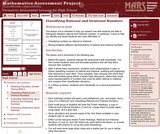Only Sharing Permitted
CC BY-NC-ND
Rating

This lesson unit is intended to help teachers assess how well students are able to distinguish between rational and irrational numbers. In particular, it aims to help teacher identify and assist students who have difficulties in: classifying numbers as rational or irrational; and moving between different representations of rational and irrational numbers.

نوع المادة:
التقييم
Lesson Plan
Provider:
Shell Center for Mathematical Education
U.C. Berkeley
Provider Set:
Mathematics Assessment Project (MAP)
04/26/2013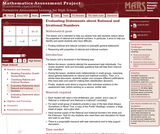Only Sharing Permitted
CC BY-NC-ND
Rating

This lesson unit is intended to help teachers assess how well students reason about the properties of rational and irrational numbers. In particular, this unit aims to help teachers identify and assist students who have difficulties in: finding irrational and rational numbers to exemplify general statements; and reasoning with properties of rational and irrational numbers.

نوع المادة:
التقييم
Lesson Plan
Provider:
Shell Center for Mathematical Education
U.C. Berkeley
Provider Set:
Mathematics Assessment Project (MAP)
04/26/2013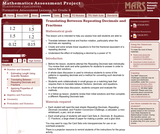Only Sharing Permitted
CC BY-NC-ND
Rating

This lesson unit is intended to help teachers assess how well students are able to: translate between decimal and fraction notation, particularly when the decimals are repeating; create and solve simple linear equations to find the fractional equivalent of a repeating decimal; and understand the effect of multiplying a decimal by a power of 10.

نوع المادة:
التقييم
Lesson Plan
Provider:
Shell Center for Mathematical Education
U.C. Berkeley
Provider Set:
Mathematics Assessment Project (MAP)
04/26/2013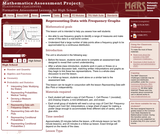Only Sharing Permitted
CC BY-NC-ND
Rating

This lesson unit is intended to help teachers assess how well students: are able to use frequency graphs to identify a range of measures and make sense of this data in a real-world context; and understand that a large number of data points allow a frequency graph to be approximated by a continuous distribution.

نوع المادة:
التقييم
Lesson Plan
Provider:
Shell Center for Mathematical Education
U.C. Berkeley
Provider Set:
Mathematics Assessment Project (MAP)
04/26/2013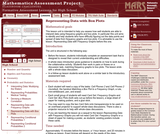Only Sharing Permitted
CC BY-NC-ND
Rating

This lesson unit is intended to help teachers assess how well students are able to interpret data using frequency graphs and box plots. In particular this unit aims to identify and help students who have difficulty figuring out the data points and spread of data from frequency graphs and box plots. It is advisable to use the lesson: Representing Data 1: Frequency Graphs, before this one.

نوع المادة:
التقييم
Lesson Plan
Provider:
Shell Center for Mathematical Education
U.C. Berkeley
Provider Set:
Mathematics Assessment Project (MAP)
04/26/2013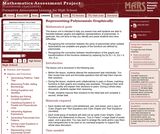Only Sharing Permitted
CC BY-NC-ND
Rating

This lesson unit is intended to help teachers assess how well students are able to translate between graphs and algebraic representations of polynomials. In particular, this unit aims to help you identify and assist students who have difficulties in: recognizing the connection between the zeros of polynomials when suitable factorizations are available, and graphs of the functions defined by polynomials; and recognizing the connection between transformations of the graphs and transformations of the functions obtained by replacing f(x) by f(x + k), f(x) + k, -f(x), f(-x).

نوع المادة:
التقييم
Lesson Plan
Provider:
Shell Center for Mathematical Education
U.C. Berkeley
Provider Set:
Mathematics Assessment Project (MAP)
04/26/2013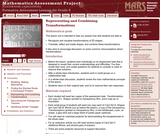Only Sharing Permitted
CC BY-NC-ND
Rating

This lesson unit is intended to help teachers assess how well students are able to: recognize and visualize transformations of 2D shapes; and translate, reflect and rotate shapes, and combine these transformations. It also aims to encourage discussion on some common misconceptions about transformations.

نوع المادة:
التقييم
Lesson Plan
Provider:
Shell Center for Mathematical Education
U.C. Berkeley
Provider Set:
Mathematics Assessment Project (MAP)
04/26/2013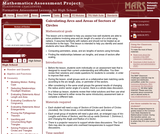Only Sharing Permitted
CC BY-NC-ND
Rating

This lesson unit is intended to help teachers assess how well students are able to solve problems involving area and arc length of a sector of a circle using radians. It assumes familiarity with radians and should not be treated as an introduction to the topic. This lesson is intended to help teachers identify and assist students who have difficulties in: Computing perimeters, areas, and arc lengths of sectors using formulas and finding the relationships between arc lengths, and areas of sectors after scaling.

نوع المادة:
التقييم
Lesson Plan
Provider:
Shell Center for Mathematical Education
U.C. Berkeley
Provider Set:
Mathematics Assessment Project (MAP)
04/26/2013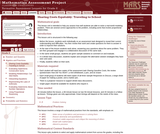Only Sharing Permitted
CC BY-NC-ND
Rating

This lesson unit is intended to help teachers assess how well students are able to solve a real-world modeling problem. There are several correct approaches to the problem, including some that involve proportional relationships.

نوع المادة:
التقييم
Lesson Plan
Provider:
Shell Center for Mathematical Education
U.C. Berkeley
Provider Set:
Mathematics Assessment Project (MAP)
Mathematics Assessment Project (MAP)
04/26/2013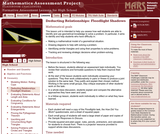Only Sharing Permitted
CC BY-NC-ND
Rating

This lesson unit is intended to help teachers assess how well students are able to identify and use geometrical knowledge to solve a problem. In particular, this unit aims to identify and help students who have difficulty in: making a mathematical model of a geometrical situation; drawing diagrams to help with solving a problem; identifying similar triangles and using their properties to solve problems; and tracking and reviewing strategic decisions when problem-solving.

نوع المادة:
التقييم
Lesson Plan
Provider:
Shell Center for Mathematical Education
U.C. Berkeley
Provider Set:
Mathematics Assessment Project (MAP)
04/26/2013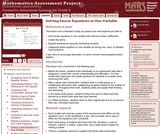Only Sharing Permitted
CC BY-NC-ND
Rating

This lesson unit is intended to help teachers assess how well students are able to: solve linear equations in one variable with rational number coefficients; collect like terms; expand expressions using the distributive property; and categorize linear equations in one variable as having one, none, or infinitely many solutions. It also aims to encourage discussion on some common misconceptions about algebra.

نوع المادة:
التقييم
Lesson Plan
Provider:
Shell Center for Mathematical Education
U.C. Berkeley
Provider Set:
Mathematics Assessment Project (MAP)
04/26/2013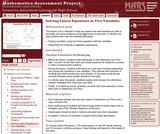Only Sharing Permitted
CC BY-NC-ND
Rating

This lesson unit is intended to help teachers assess how well students are able to formulate and solve problems using algebra and, in particular, to identify and help students who have the following difficulties: solving a problem using two linear equations with two variables; and interpreting the meaning of algebraic expressions.

نوع المادة:
التقييم
Lesson Plan
Provider:
Shell Center for Mathematical Education
U.C. Berkeley
Provider Set:
Mathematics Assessment Project (MAP)
04/26/2013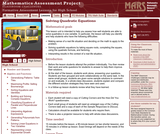Only Sharing Permitted
CC BY-NC-ND
Rating

This lesson unit is intended to help teachers assess how well students are able to solve quadratics in one variable. In particular, the lesson will help teachers identify and help students who have the following difficulties: making sense of a real life situation and deciding on the math to apply to the problem; solving quadratic equations by taking square roots, completing the square, using the quadratic formula, and factoring; and interpreting results in the context of a real life situation.

نوع المادة:
التقييم
Lesson Plan
Provider:
Shell Center for Mathematical Education
U.C. Berkeley
Provider Set:
Mathematics Assessment Project (MAP)
04/26/2013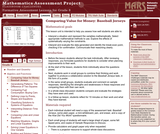Only Sharing Permitted
CC BY-NC-ND
Rating

This lesson unit is intended to help teachers assess how well students are able to: interpret a situation and represent the variables mathematically; select appropriate mathematical methods to use; explore the effects of systematically varying the constraints; interpret and evaluate the data generated and identify the break-even point, checking it for confirmation; and communicate their reasoning clearly.

نوع المادة:
التقييم
Lesson Plan
Provider:
Shell Center for Mathematical Education
U.C. Berkeley
Provider Set:
Mathematics Assessment Project (MAP)
04/26/2013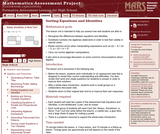Only Sharing Permitted
CC BY-NC-ND
Rating

This lesson unit is intended to help teachers assess how well students are able to: recognize the differences between equations and identities; substitute numbers into algebraic statements in order to test their validity in special cases; resist common errors when manipulating expressions such as 2(x Đ 3) = 2x Đ 3; (x + 3)_ = x_ + 3_; and carry out correct algebraic manipulations. It also aims to encourage discussion on some common misconceptions about algebra.

نوع المادة:
التقييم
Lesson Plan
Provider:
Shell Center for Mathematical Education
U.C. Berkeley
Provider Set:
Mathematics Assessment Project (MAP)
04/26/2013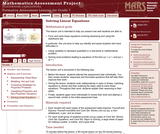Only Sharing Permitted
CC BY-NC-ND
Rating

This lesson unit is intended to help teachers assess how well students are able to: form and solve linear equations involving factorizing and using the distributive law. In particular, this unit aims to help teachers identify and assist students who have difficulties in: using variables to represent quantities in a real-world or mathematical problem and solving word problems leading to equations of the form px + q = r and p(x + q) = r.

نوع المادة:
التقييم
Lesson Plan
Provider:
Shell Center for Mathematical Education
U.C. Berkeley
Provider Set:
Mathematics Assessment Project (MAP)
04/26/2013Only Sharing Permitted
CC BY-ND
Rating

إن رسالة "فهم العلوم" هو أن يقدم مورد ممتع وسهل الوصول ومجاني يعمل على التعريف الدقيق بماهية العلوم وكيفية عملها فعلاً. إن المنهج العلمي شيق، إلا أن الشروحات القياسية غالباً ما تغفل عن الطبيعة الديناميكية للعلوم. وتؤثر العلوم فينا جميعاً كل يوم، لكن كثيراً ما يشعر الناس بأنهم منعزلون عن العلوم. إن العلوم هي مسعى إنساني مكثف، إلا أن العديد من التصويرات تخفي جوانب الشغف والفضول، بل وحتى المنافسات والمكائد، التي تميز كل المشروعات الإنسانية. يقدم "فهم العلوم" للمستخدمين نظرة من الداخل على المبادئ العامة والمناهج والمحفزات التي تندرج في كل العلوم. يتضمن هذا المشروع في رحمه محاولة لإعادة الاندماج مع العلوم، والتي تبدأ بإعداد المعلم وتنتهي بالفهم العام الأوسع للعلوم. وتضم الأهداف العاجلة للمشروع: (1) تحسين فهم المعلم لطبيعة العمل العلمي، (2) توفير الموارد والاستراتيجيات التي تشجع معلمي الصفوف من الحضانة وحتى السادس عشر على تدعيم طبيعة العلوم طوال ممارستهم لتدريس العلوم، (3) توفير مرجع واضح وغزير للطلاب وعموم الناس، والذي يصور المسعى العلمي بدقة. لقد جرى إنشاء موقع "فهم العلوم" بواسطة متحف متحجرات جامعة كاليفورنيا بمدينة بيركلي، وذلك بالتعاون مع مجموعة متنوعة من العلماء والمعلمين، وتم تمويله بواسطة المؤسسة الوطنية للعلوم1. علماً بأن "فهم العلوم" كان قد استلهم واستوحى فكرته في البداية من عملنا المتعلق بمشروع "فهم التطور" الذي أبرز حقيقة أن العديد من المفاهيم المغلوطة حول التطور تنبع من سوء فهم طبيعة العلوم. فضلاً عن ذلك، يشير البحث إلى أن الطلاب والمعلمين في كل المستويات الدراسية لديهم فهم منقوص لطبيعة ومنهج العلوم، الأمر الذي يمكن أن نعزوه إلى الفصول المدرسية حيث يتم فيها تدريس العلوم بوصفها عملية بسيطة وخطية ولا تتولد عنها أشياء. وهذا التصوير الكاذب والمُقفِر يجعل الطلاب ينفصلون عن العلوم، ويثبط الدعم العام لها، وربما يساعد على تفسير المؤشرات الحالية بأن الولايات المتحدة تفقد ثقلها العالمي في مجال العلوم. فحتى وأبعد من الاهتمام بصحة الاقتصاد الأميركي، فإن عامة الناس بداخلهم احتياج أصيل للتقييم النقدي للطروحات المتضاربة للدليل العلمي في وسائل الإعلام. ولكي يفعلوا هذا، عليهم فهم مواطن القوة والقيود والمناهج الأساسية للمشروع الذي أنتج تلك الادعاءات العلمية. يقوم "فهم العلوم" بخطوة هامة نحو الوفاء بهذه الاحتياجات.

نوع المادة:
Activity/Lab
Diagram/Illustration
Homework/Assignment
تفاعلية
ملاحظات المحاضرة
Lesson Plan
القراءة
Teaching/Learning Strategy
Provider:
AMSER: Applied Math and Science Education Repository
Internet Scout Project
U.C. Berkeley
المؤسسة الجامعية لأبحاث الغلاف الجوي
Provider Set:
AMSER: Applied Math and Science Education Repository
Internet Scout Project
المؤلفون الأفراد
DLESE Community Collection
المؤلف:
Individual Authors
02/16/2011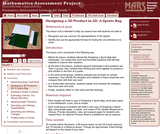Only Sharing Permitted
CC BY-NC-ND
Rating

This lesson unit is intended to help you assess how well students are able to: recognize and use common 2D representations of 3D objects and identify and use the appropriate formula for finding the circumference of a circle.

نوع المادة:
التقييم
Lesson Plan
Provider:
Shell Center for Mathematical Education
U.C. Berkeley
Provider Set:
Mathematics Assessment Project (MAP)
المؤلف:
http://map.mathshell.org/
04/26/2013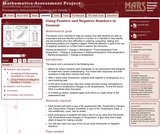Only Sharing Permitted
CC BY-NC-ND
Rating

This lesson unit is intended to help teachers assess how well students are able to understand and use directed numbers in context. It is intended to help identify and aid students who have difficulties in ordering, comparing, adding, and subtracting positive and negative integers. Particular attention is paid to the use of negative numbers on number lines to explore the structures: starting temperature + change in temperature = final temperature final temperature Đ change in temperature = starting temperature final temperature Đ starting temperature = change in temperature.

نوع المادة:
التقييم
Lesson Plan
Provider:
Shell Center for Mathematical Education
U.C. Berkeley
Provider Set:
Mathematics Assessment Project (MAP)